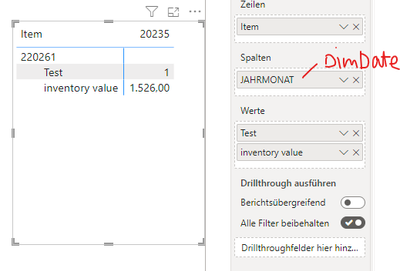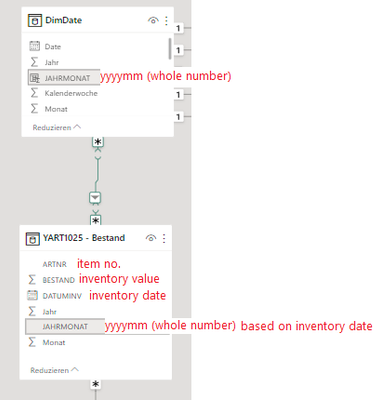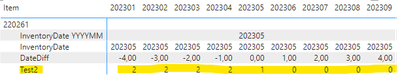cancel
Showing results for
Did you mean:

Fabric is Generally Available. Browse Fabric Presentations. Work towards your Fabric certification with the Cloud Skills Challenge.Frequent Visitor

## Compare date from fact table with date in matrix with dax measure

Hi everyone,

I am having trouble with the following issue:

• I have a date table called 'DimDate' and a table with inventory information 'YART1025 - Bestand' for individual items and an inventory date.
• Example: The item '220261' has an inventory value of 1,526 pieces on 2023/05/02 (second may 2023).
• What I did: I created a matrix visual as following:
 DimDate[Date] Article Measure [Test]

• I then created a measure called [Test] with an IF statement that if the inventory date matchs with the date in the matrix column, the value 1 is output:

``````Test =
var _InventoryDate = MAX('YART1025 - Bestand'[JAHRMONAT])
var _MatrixDate = SELECTEDVALUE(DimDate[JAHRMONAT])

RETURN
IF(
_inventoryDate = _MatrixDate,
1,
blank()
)​``````

This has worked so far:However, if I change this measure to the following condition, no correct values come out. What could be the reason for this?

``````Test =
var _InventoryDate = MAX('YART1025 - Bestand'[JAHRMONAT])
var _MatrixDate = SELECTEDVALUE(DimDate[JAHRMONAT])

RETURN
IF(
_inventoryDate < _MatrixDate,
1,
blank()
)``````The matrix shows results up to year 2000, even though the inventory date is in 2023 and is bigger than the date in the column of the matrix, so I'm expecting a blank result here. Same happens, if I change the measure in _inventoryDate > _MatrixDate. Every column gets a '1' as a result except the column 20235, where _inventoryDate = _MatrixDate.

Data model:Anett

*****EDIT

I tried to clear some data types (its all decimals now) and re-wrote the measure into following (don't worry about the new column reference "YYYYMM" in the table DimDate):

``````Test =
var _InventoryDate = MAX('YART1025 - Bestand'[JAHRMONAT])
var _MatrixDate = SELECTEDVALUE(DimDate[YYYYMM])

RETURN
SWITCH(
TRUE(),
_InventoryDate = _MatrixDate, 1,
_InventoryDate < _MatrixDate, 0,
_InventoryDate > _MatrixDate, 2,
blank()
)``````

The result looks like this:So this measure shows, that the date in Matrix is always bigger than the inventory date - except in the month of the inventory. So at least, this part is correct. I really don't understand this result.

1 ACCEPTED SOLUTIONFrequent Visitor

For anyone who stumbles across the same issue - that gave me the solution:

Because there was no inventory date in the later/sooner month columns of the matrix, the DAX formula had to compare a blank value (inventory date) with the matrix date. That has led to the result that the comparison in the DAX formula has determined that the inventory date is less than the matrix date.

What I did: I rewrote the DAX for identifying the inventory date like this:

``````_InventoryDate =
CALCULATE(
MAX('YART1025 - Bestand'[JAHRMONAT]),
REMOVEFILTERS(DimDate2)
)``````

As a result, the inventory date is being displayed for every column of the matrix regardless of the matrix date. Like that, the comparison of both dates can be performed:

``````Test2 =
var _InventoryDate =
CALCULATE(
MAX('YART1025 - Bestand'[JAHRMONAT]),
REMOVEFILTERS(DimDate2)
)
var _MatrixDate = SELECTEDVALUE(DimDate2[YYYYMM])

RETURN
SWITCH(
TRUE(),
_MatrixDate = _InventoryDate, 1,
_MatrixDate > _InventoryDate, 0,
_MatrixDate < _InventoryDate, 2,
blank()
)``````

Result:4 REPLIES 4Frequent Visitor

For anyone who stumbles across the same issue - that gave me the solution:

Because there was no inventory date in the later/sooner month columns of the matrix, the DAX formula had to compare a blank value (inventory date) with the matrix date. That has led to the result that the comparison in the DAX formula has determined that the inventory date is less than the matrix date.

What I did: I rewrote the DAX for identifying the inventory date like this:

``````_InventoryDate =
CALCULATE(
MAX('YART1025 - Bestand'[JAHRMONAT]),
REMOVEFILTERS(DimDate2)
)``````

As a result, the inventory date is being displayed for every column of the matrix regardless of the matrix date. Like that, the comparison of both dates can be performed:

``````Test2 =
var _InventoryDate =
CALCULATE(
MAX('YART1025 - Bestand'[JAHRMONAT]),
REMOVEFILTERS(DimDate2)
)
var _MatrixDate = SELECTEDVALUE(DimDate2[YYYYMM])

RETURN
SWITCH(
TRUE(),
_MatrixDate = _InventoryDate, 1,
_MatrixDate > _InventoryDate, 0,
_MatrixDate < _InventoryDate, 2,
blank()
)``````

Result:Helper I

The measure is correct. I think the data in your table doesn't fit the measure. Tip: try chatGPT if you search for answers.Helper I

The Inventorydate is written with a "i" and should be "I", this could be the problem.Frequent Visitor

thanks for your reply! Unfortunately, after correcting the "I" the calculation is still wrong.Announcements#### Power BI Monthly Update - November 2023

Check out the November 2023 Power BI update to learn about new features.#### Fabric Community News unified experience

Read the latest Fabric Community announcements, including updates on Power BI, Synapse, Data Factory and Data Activator.#### Exclusive opportunity for Women!

Join us for a free, hands-on Microsoft workshop led by women trainers for women where you will learn how to build a Dashboard in a Day!#### The largest Power BI and Fabric virtual conference

130+ sessions, 130+ speakers, Product managers, MVPs, and experts. All about Power BI and Fabric. Attend online or watch the recordings.Top Solution Authors
Top Kudoed Authors
Users online (4,739)